# topic diversity介绍

Joseph A. Konstan教授(coursera.明大.推荐系统课程)在2005年《Improving Recommendation Lists Through Topic Diversification》中提出了主题多样性。虽然这篇paper比较老，但比较经典，值得一看：

# 1.介绍

## 1.1 贡献

• 主题多样性(topic diversification)：我们提出了一种方法，根据该活跃用户的完整范围的兴趣，来平衡top-N推荐列表。我们的新方法会同时考虑：suggestions给出的accuracy，以及在特定主题上的用户兴趣范围。主题多样性的分析包含：user-based CF和item-based CF.
• Intra-list相似度指标（similarity metric）：
• accuracy vs. satisfaction：

# 3.评估指标

## 3.1 Accuracy Metrics

Accuracy metrics主要有两个：

## 3.2 Accuracy之外

### 3.2.2 Noverlty和Serendipity

Novelty和serendipity指标可以衡量推荐的”非显而易见性（non-obviousness）”，避免“随机选取（cherry picking）”。对于serendipity的一些简单measure，可以采用推荐items的平均流行度。分值越低表示越高的senrendipity。

## 3.3 Intra-List Similarity

$ILS(P_{w_i}) = \frac{\sum\limits_{b_k \in \Im P_{w_i}} \sum\limits_{b_e \in \Im P_{w_i}, b_k \neq b_e} c_o(b_k, b_e)}{2}$

…(5)

$\forall \delta_i, \delta_j \in S_N: ILS(P_{w_i} \circ \delta_i) = ILS(P_{w_i} \circ \delta_j)$

…(6)

# 4.topic diversification

acurracy指标的一个主要问题是，它不能捕获更宽泛的用户满意度，会隐藏在已存在系统中的一些明显缺陷。例如，推荐出一个非常相似items的列表（比如：对于一个很少使用的用户，根据author、genre、topic推出），尽管该列表的平均accuracy可能很高。

## 4.1 Taxonomy-based Similarity指标

classification taxonomies存在不同领域。Amazon.com为books/DVSs/CDs/电子产品等制作了非常大的taxonomies。图1表示一个sample taxonomy。另外，在Amazon.com上的所有商品的内容描述与它们的domain taxonomies相关。特征化的主题可以包含：author、genre、audience。## 4.2 topic diversification算法## 4.4 渗透压（Osmotic Pressure）类比

dissimilarity效应与分子生物学上的渗透压和选择性渗透（selective permeability）相类似。将商品$b_o$（它来自兴趣$d_o$的特定领域）稳定插入到推荐列表中，等价于：从来自一个特定物质的分子通过细胞膜传到细胞质中。随着浓度$d_o$（它属于膜的选择性通透性）的增加，来自其它物质d的分子b的压力会上升。对于一个给定主题$d_p$，当压力(pressure)足够高时，它最好的商品$b_p$可能“散开（diffuse）“到推荐列表中，尽管他们的原始排序（original rank）$P_{w_i}^{-1}(b)$可能不如来自流行域（prevailing domain）$d_o$。相应的，对于$d_p$的压力会下降，为另一个压力上升的domain铺路。

# 3.QuadRank

QuadSearch的缺省ranking fusion算法是一个与位置有关（positional）的方法，可以处理partial ranked list；实际上它会处理来自基于single-crawler的搜索引擎们的top-k lists。我们这样做的原因是因为以下普通并且显著的观察：

• i) 所有这些都被experts认为是“major”搜索引擎s
• ii)他们在lifetimes期间是可靠的
• ii)大多数用户和metasearch engines更偏向于他们来执行它们的搜索## 3.1 处理individual rankings

$K(c)=\sum\limits_{i=1}^m (k+1 - r_i(c))$

…(2)

• m: 表示所使用的component engines的数目
• c: 表示在component list中的单个结果
• k: 在每个component list中的items数目
• $r_i(c)$是该item被分配给第i个component engine得到的ranking• 1.$c_2$在更多的input rankings中出现，相应的，根据民主对称性(democratic symmetry) Saari(2000)
• 2.$c_2$在超过一半的input rankings中出现，因此根据孔多塞标准（Condorcet criterion），它是一个spam entry的概率很低

$R(c)=m log(n_c K(c))$

…(3)

# 1.介绍# 3.方法

## 3.1 低维商品embeddings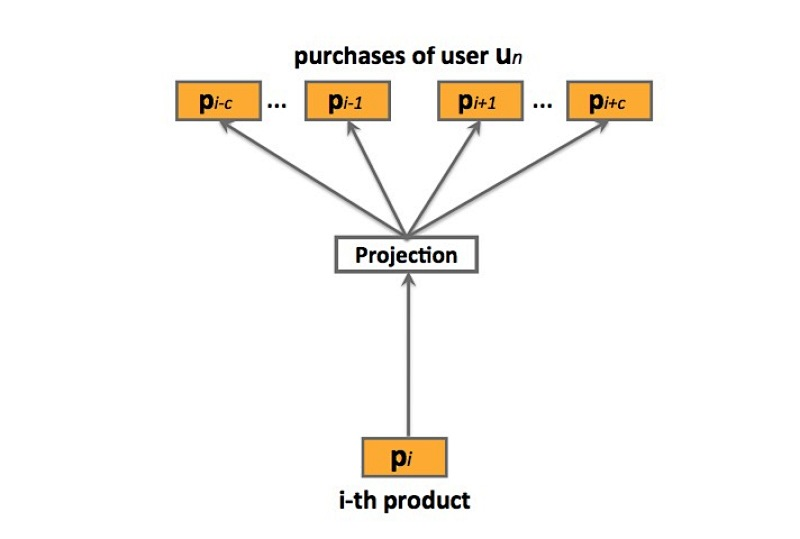prod2vec：prod2vec模型会将一个购买序列看成是一个“句子”，在这个序列中的商品看成是“词”。详见图2, 更特殊的，prod2vec使用skip-gram模型来学习商品表示，通过以下的最大化目标函数：

$L = \sum_{s \in S} \sum_{p_i \in s} \sum_{-c \leq j \leq c, j \neq 0} log p(p_{i+j} | p_i)$

…(3.1)

$P(p_{i+j}| p_i) = \frac{exp({v_{p_i}^T v_{p_{i+j}}'})} {\sum_{p=1}^P exp{(v_{p_i}^T v_p')}}$

…(3.2)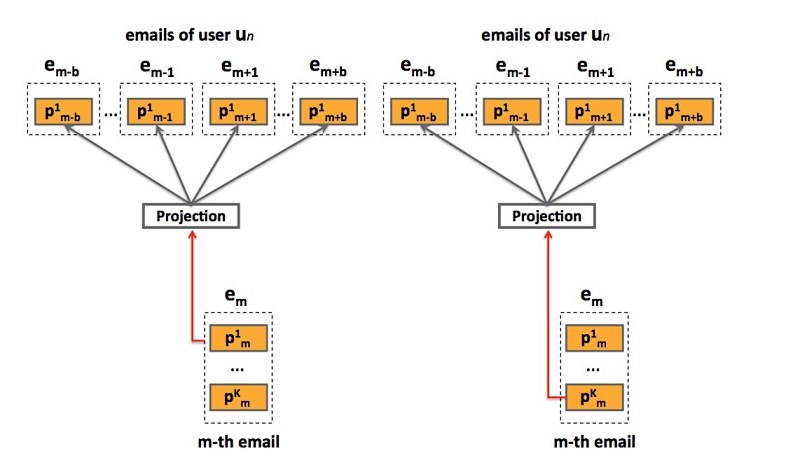bagged-prod2vec：为了对多个商品同时购买的行为做出解释，我们提出了一个修改版本的skip-gram模型，它引入了一个概念：购物袋（shopping bag）。如图3所示，该模型会在email receipts级别进行操作，而非在商品级别。通过对email序列s上的一个修改版目标函数进行最大化，来学习商品向量表示：

$L = \sum_{s \in S} \sum_{e_m \in s} \sum_{-n \leq j \leq n, j\neq 0} \sum_{k=1,...,T_m} log P(e_{m+j}| p_{mk})$

…(3.3)

$P(e_{m+j} \mid p_{mk}) = P(p_{m+j,1} \mid p_{mk} ) \times ... \times P(p_{m+j,T_m} \mid p_{mk})$

learning：该模型使用SGA（ stochastic gradient ascent）进行最优化，很适合大规模问题。然而，在(3.1)和(3.3)中的梯度计算$\Delta L$，很适合词汇size P，实际任务中，计算开销会随着P的增大而变得很昂贵，很容易达到上百万的商品。另一方面，我们使用negative sampling方法，它能显著减小计算复杂度。

## 3.2 prod-2-prod预测模型

prod2vec-topK：给定一个购买商品，该方法会为所有在词汇表中的商品计算cosine相似度，并推荐最相似商品的top K

prod2vec-cluster：为了能做出更多样化(diverse)的推荐，我们考虑将相似商品分组成聚类，从与之前购买商品相关的聚类中推荐商品。我们应用K-means聚类算法来在hadoop FS上实现，将商品基于cosine相似度进行聚类。我们假设：在从聚类$c_i$上进行一个购买后、再从任意第C个聚类中进行一次购买的行为，符合一个多项式分布（multinomial distribution）$M_u(\theta_{i1}, \theta_{i2}, ..., \theta_{iC})$，其中$\theta_{ij}$是从聚类$c_i$中进行一次购买后、接着从聚类$c_j$中进行一次购买的概率。为了估计参数$\theta_{ij}$，对于每个i和j，我们采用一个最大似然方法：

$\hat {\theta_{ij}} = \frac{c_i购买后跟c_j的次数}{c_i购买的总数}$ …(3.4)

• count of ci purchases: c_i购买的数目
• # of times ci purchase was followed by cj: c_i购买后跟c_j的次数

## 3.3 User-to-product预测模型

user2vec：受paragraph2vec算法的启发，user2vec模型会同时学习商品和用户的特征表示，它会将用户当成是一个“全局上下文”。这样的模型如图4所示。训练数据集来自于用户购买序列S，它会包含$u_n$和其它已购商品（通过购买时间序排列），$u_n = (p_{n1}, p_{n2}, ..., p_{nU_n})$，其中$U_n$表示用户$u_n$购买的items数目。在训练期间，用户向量会被更新，来预测从他的email receipts中的商品，其中，学到的product vectors会预测在上下文中的其它商品。出于表示的简洁性，在下面，我们会表示no-bagged版本的模型，注意，使用bagged版本进行扩展也很方便。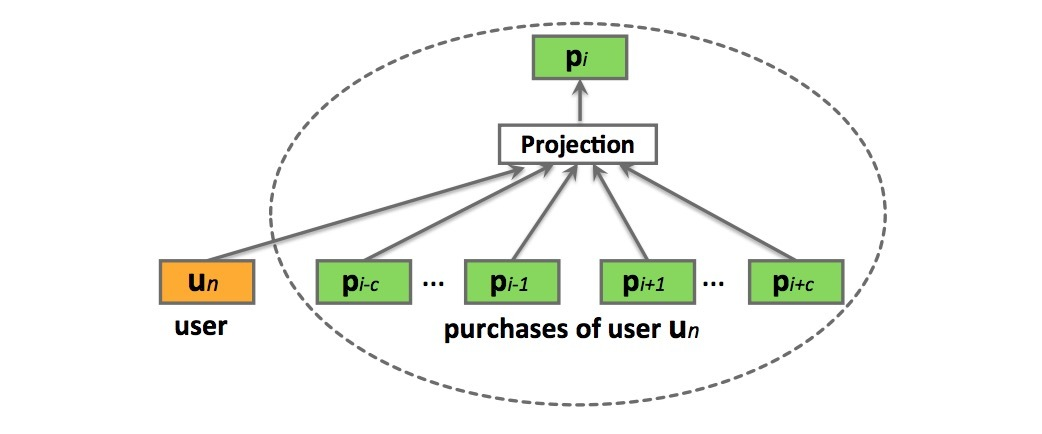$L = \sum_{s \in S} (\sum_{u_n \in s} log P(u_n | p_{n1}: p_{nU_n}) + \sum_{p_{n_i} \in u_n} log P(p_{ni}| p_{n,i-c}: p_{n,i+c,u_n}))$

…(3.5)

$P(p_{ni}|p_{n,i-c}: p_{n,i+c}, u_n) = \frac{e^{\bar{v}^T v_{p_{ni}}'}}{ \sum_{p=1}^{V} e^{\bar{v}^T v_p'}}$ …(3.6)

$\bar{v} = \frac{1}{2c+1} (v_{u_n} + \sum_{-c \leq j \leq c ,j \neq 0} v_{p_{n,i+j}})$ …(3.7)

$P(u_n | p_{n1}: p_{nU_n}) = \frac{e^{\bar{v}_n^T v_{u_n}'}} {\sum_{p=1}^V e^{\bar{v}_n^T v_p'}}$ …(3.8)

$\bar{v}_n = \frac{1}{U_n} \sum_{i=1}^{U_n} v_{p_{ni}}$

…(3.9)

user2vec模型的一个主要优点是，商品推荐是基于该用户的购买历史进行量身定制的。然而，缺点是，该需要需要非常频繁地进行更新，不同于product-to-product方法，它可以长期是相关的，而user-to-product推荐需要经常变化来对最近购买行为做出解释。

# 4.实验及其它

## 4.4 推荐预测商品

• 1) prod2vec-topK：使用数据集$D_p$进行训练，其中，商品向量通过对购买序列s通过极大似然估计进行学习。给定一个商品$p_i$，通过向量空间计算cosine相似度选择topK个相似商品。
• 2) bagged-prod2vec-topK：使用$D_p$进行训练，其中商品向量通过email序列s通过极大似然估计进行学习。对于给定商品$p_i$，通过选择在结合向量空间计算cosine相似度选择topK个相似商品。
• 3) bagged-prod2vec-cluster: 与bagged-prod2vec模型的训练类似，接着将商品向量聚类成C个聚类，并计算它们间的转移概率。接着标识出$p_i$属于哪个聚类（例如：$p_i \in c_i$），我们根据$c_i$的转移概率对各聚类进行排序，取出top个聚类，然后聚出这些top聚类的商品通过计算与$p_i$的cosine相似度进行排序，其中每个聚类的top $K_c$被用于推荐（$\sum {K_c} = K$）。 bagged-prod2vec-cluster与 bagged-prod2vec的预测结果如表2所示。可以看到聚类方法多样性更好。
• 4) user2vec：使用$D_p$进行训练，其中商品向量和用户向量通过极大似然估计进行学习。给定一个用户$u_n$，通过计算$u_n$用户向量与所有商品向量的cosine相似度，检索出top K近邻商品。
• 5) co-purchase：对于每个购买pair：$(p_i, p_j)$，计算频率$F_{(p_i,p_j)}$，其中$i=1,...,P, j=1,...,P$，商品$p_j$在商品$p_i$之后立即购买。接着，给商品$p_i$的推荐通过频率$F_{(p_i,p_j)}, j=1,...,P$进行排序，取topK商品。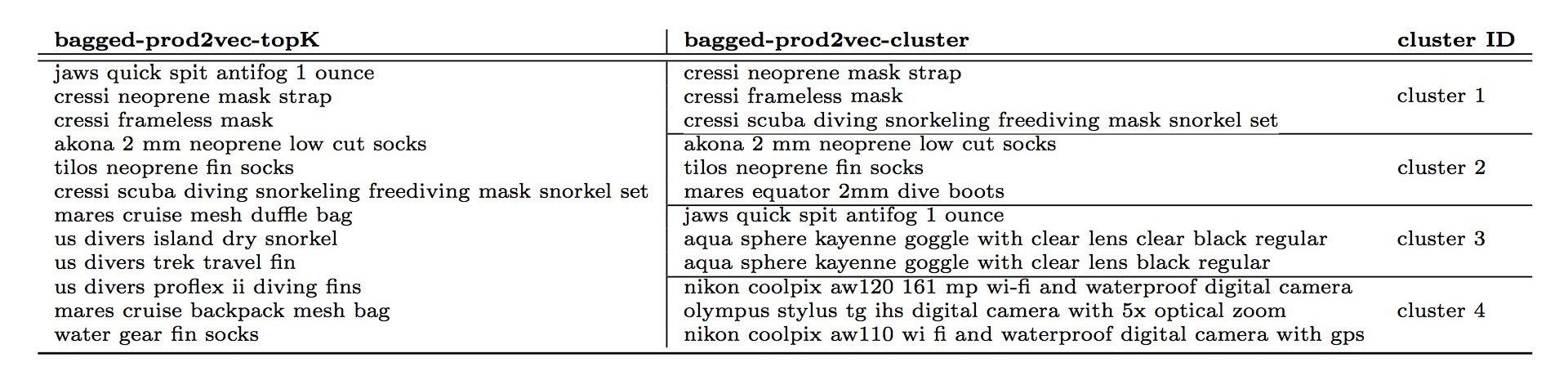$d_j = sim_j \cdot \alpha^{t_d - t_i}$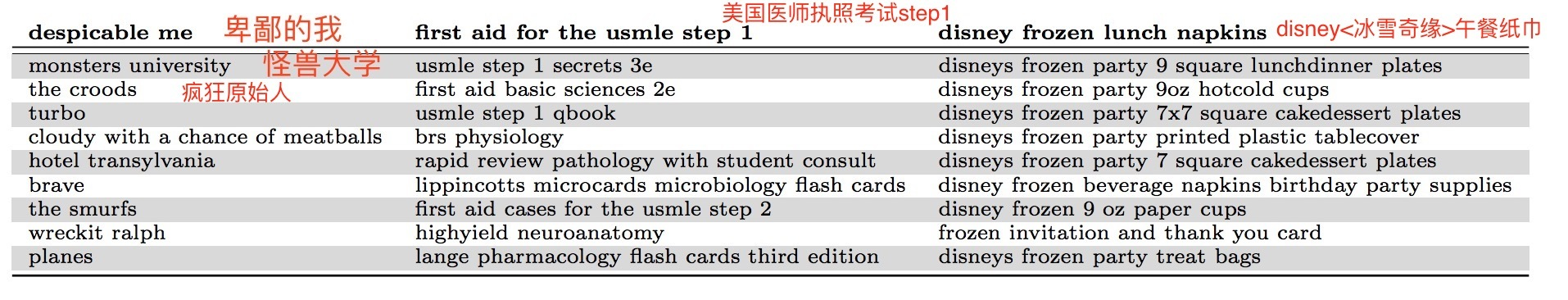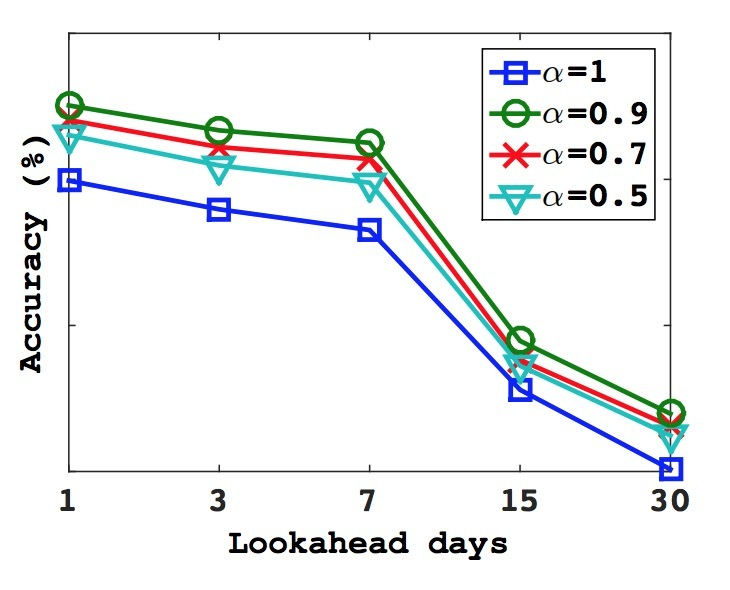# 1.介绍

## 2.相关工作

NLP的字符级特征（Character level features）：与我们工作相关的另一个研究领域是，NLP的字符级模型，它直接从字符序列中学习表示。这种模型的最高级方法是RNN，应用到语言建模（Mikolov 2012）、文本归一化（Chrupala, 2014）,、词性标注(Ling.2015)、parsing（Ballesterors.2015）。该模型的另一个家族是在字符上训练的CNN，它可以被应用到：词性标注（Santos,2014）、情感分析（dos Santos.2014）、文本分类（zhang.2015）、语言建模（Kim.2016）。(Sperr.2013)提出了基于受限波尔茨曼机的语言模型，其中词被编码成字符级别n-grams的一个集合。最后，在机器翻译中的最近工作也提出了使用subword units来获取罕见词的表示。（Sennrich.2016）

# 3.模型

## 1.1 通用模型

$\sum_{t=1}^{T}\sum_{c \in {C_t}}log p(w_c|w_t)$

$p(w_c|w_t)=\frac{e^{s(w_t,w_c)}}{\sum_{j=1}^{W}e^{s(w_t,j)}}$

$log(1+e^{-s(w_t,w_c)})+\sum_{n \in {N_{t,c}}} log(1+e^{s(w_t,n)})$

$\sum_{t=1}^{T}\sum_{c \in C_t}l(s(w_t,w_c))+\sum_{n \in N_{t,c}}l(-s(w_t,n))$

$w_t$和上下文词$w_c$采用标量积：$s(w_t,w_c)=u_{w_t}^{T}v_{w_c}$

## 1.2 Subword模型

$s(w,c)=\sum_{g \in {G_w}}z_g^Tv_c$

# 1.4 试验

• 小数据集：100维, 中数据集：200维，完整数据集:300维.
• skip-gram baseline learning-rate: 0.025; CBOW: 0.05, 新模型:0.05

• 英文：使用 WS353 (Finkelstein et al.2001)以及 RW (Luong et al.2013)
• 德文：Gur65, Gur350,ZG222(Gurevych, 2005; Zesch and Gurevych, 2006）
• 法文：RG65(Joubarne and Inkpen, 2011)
• 西班牙文：WS353(Hassan and Mihalcea, 2009)# 2.高效文本分类tricks

Mikolov等在中提到了多种高效文本分类的tricks，并提出了fastText。它的分类速度快，又不失精准。在标准多核CPU上训练，超过10亿词上只需要10分钟左右；而对50w的句子，在312K个分类上进行分类，1分钟之内即可完成。听上去有些小激动。

## 2.1 模型架构

baseline: 对于句子分类，简单又有效的baseline为：BOW向量 + 一个线性分类器(LR或SVM)。$-\frac{1}{N}\sum_{n=1}^{N}y_nlog(f(BAx_n))$

### Hierarchical softmax

$P(n_{l+1})=\prod_{i=1}^{l}P(n_i)$

### N-gram features

BOW与词序无关，显式采用该顺序的计算开销通常很大。作为替代，我们使用bag-of-n-grams作为额外的特征来捕获一些关于局部词序的部分信息(partial information)。这在惯例上很有效（Wang and Manning, 2012).

## 2.2 实验评测

fastText在两个不同的任务上进行评测。首先，会比较在情感分析（sentiment analysis）问题上的文本分类器。模型的实现可以使用Vowpal Wabbit library，但实际上使用的定制版本要比它快2-5x倍。

### 情感分析(Sentiment analysis)### 标签预测# 介绍

• X是一个任意输入空间
• $A=\lbrace 1, \cdots, k \rbrace$是一个actions集合

• 1.抽取$(x, \vec{r}) \sim D$，并公布x
• 2.算法会选择一个action $a \in A$，可以是一个关于x和历史信息的函数
• 3.公布action a的reward为$r_a$，但不会公布$a'\neq a$是$r_{a'}$

$V^h := \underset{(x,r) \sim D}{E} [r_{h(x)}]$

• 1.监督学习：我们可以学到一个regressor $s: X \times A \rightarrow [0,1]$，它可以在观察到的events上基于action a和其它信息x，训练来预测reward。从该regressor，一个policy可以根据$h(x)=argmax_{a \in A} s(x,a)$来生成。该方法的缺点是，argmax会超出在训练数据中未包含的选择集合，因此不能被泛化。这可以通过一些极端情况进行验证。假设，有两个actions a和b，其中action a出现$10^6$次，action b出现$10^2$次。由于action b的次数比例只占$10^{-4}$，一个学习算法会强迫在预测$r_a$和$r_b$的期望值间做权衡，并压倒性地偏向于估计$r_a$，代价是精准估计$r_b$。然而，在应用上，action b可能会被argmax选中。当action b出现0次时，该问题会更糟糕
• 2.Bandit方法：略
• 3.Contextual Bandits：略
• 4.Exploration Scavenging：略
1. 倾向指数（Propensity Scores）, naively：当进行一个调查时，提问一个关于收入的问题，接着在不同收入级别上的回答者的比例，可以与基于参与该调查所选中的某些人的收入估计一个条件概率进行对比。给定该估计概率，结果可以被importance-weighted来估计在整个人口(population)上的平均调查结果。这里，该方法是有问题的，因为policy会做出决策，当记录数据是deterministic而非probabilistic。换句话说，对logging policy选择一个ad的概率的精准预测，意味着总是预测0或1, 这对于我们的目标是没用处的。尽管propensity scores的直接使用并不work，我们采用的该方法可以被认为是，对于一个propensity score的更好使用，下面会讨论。Lambert提供了一个在互联网ad环境中关于propensity scoring的一个良好解释。

• 1.对于每个event $(x,a,r_a)$，使用回归(regression)来估计logging policy会选择action a的概率(probability) 为$\hat{\pi}(a \mid x)$。这里，“probability”是随时间变化的——我们可以想像：在不同时间点，采用一个均匀随机的方式从policies集合（可能是deterministic）中抽取。
• 2.对于每个event $(x,a,r_a)$，根据$(x,a,r_a, \frac{1}{max \lbrace \hat{\pi}(a \mid x), \tau \rbrace})$，创建一个synthetic controlled contextual bandit event，其中$\tau > 0$是一些参数。$\frac{1} { max \lbrace \hat{\pi}(a \mid x), \tau \rbrace}$这个量是一个importance weight，它指定了当前event对于训练是有多重要。需要明确的是，参数$\tau$对于数值稳定性很重要。
• 3.应用一个offline contextual bandit算法到synthetic contextual bandit events集合上。在我们第二个实验结果的集合（4.2节）中，采用的argmax regressor的变种使用了两个关键修改之处：(a) 我们将argmax的范围限定在具有正概率的那些actions上 (b) 我们对events进行importance weight，以便训练过程会强调对于每个action平等的好估计。需要强调的是，在本paper中的理论分析可以应用于对于在contextual bandit events学习上的任何算法——我们选择该方法是因为它很容易在已存在方法上进行简单修改。

• 1.在当给定特征x时，logging policy会确定式(deterministically)选中一个action (ad) a，$\hat{\pi}(a \mid x)$意味着什么，？基本observation：一个policy如果在day 1会确定式选中action a、接着在day 2会确定选中action b，这可以被看成是：(当events数目在每天都相同时，并且events间是IID的），在action a和b间使用概率0.5进行随机化。因而，在logged events的时间跨度上，$\hat{\pi}(a \mid x)$是一个给定特征x关于action a被展示的期望频率的估计。第3节中，我们会展示该方法在期望上是合理的，它提供了关于new policy的值的一种无偏估计。
• 2.在$\hat{\pi}(a \mid x)$上的客观误差（inevitable errors）是如何影响该过程的？结果表明它们有影响，取决于$\tau$。对于非常小的$\tau$值，$\hat{\pi}(a \mid x)$的估计必须极其精准来生成好的效果；而对于更大的值，会需要更小的accuracy。第3.1节会证明健壮性
• 3.参数$\tau$是如何影响最终结果的？当在估计过程中创建一个bias时，结果表明该bias的形式是很轻微的并且相对合理的——基于条件x具有低频展示的actions具有一个under-estimated value。这与期望的对于没有频率的actions的限制是一致的。

# 2.问题设定和假设

• $\pi_1, \cdots, \pi_T$是T个policies，

• $(x,r)$按第1节所述的方式从D中抽取
• action $a \sim \pi_t(x)$根据第t个policy被选中。

offline policy estimator

$S = \lbrace (x_t, a_t, r_{t,a_t}) \rbrace_{t=1}^T$

…(1)

$\hat{V}_{\hat{\pi}}^h(S) = \frac{1}{|S|} \sum\limits_{(x,a,r) \in S} \frac{r_a I (h(x)=a)}{max \lbrace \hat{\pi}(a \mid x), \tau \rbrace}$

…(2)

# 3.理论成果

• 1.我们具有一个policy estimation阶段，其中我们估计未知的logging policy;
• 2.我们具有一个policy optimization阶段，其中我们会使用我们估计的logging policy。

logging policy $\pi_t$可能是确定的(deterministic)，这意味着：基于logging policy中的随机化的常用方法是不可用的。我们会在下面展示：当现实情况是IID条件、并且policy会随actions的不同而变化时，这是ok的。我们会有效使用算法中的随机化标准方法来替代现实中的随机

$\pi(a | x) = \underset{t \sim UNIF(1,\cdots,T)}{E} [\pi_t (a | x)]$

…(3)

stochastic policy $\pi$会在T policies $\pi_t$上随机均匀选择一个action。我们的第一个结果是，当现实中根据$\pi$或者policies序列$\pi_t$进行选择actions时，我们的estimator的期望值与是相同的。尽管该结果和它的证明是简单的，它是本paper后面部分的基础。注意，policies $\pi_t$可以是任意的，但我们会假设，它们不依赖于用于evaluation的数据。该假设只对证明来说是必要的，可以在实际中放宽些，如4.1节。

$E_{S \sim (D,\pi_i(\cdot|x))_{i=1}^T} \hat{V}_{\hat{\pi}}^h(S) = E_{(x,\vec{r}) \sim D, a \sim \pi(\cdot |x)} \frac{r_a I (h(x)=a)}{max \lbrace \hat{\pi}(a|x), \tau \rbrace}$

…(4)

S就是上面指的dataset。

## 3.1 Policy Estimation

$reg(x)= \underset{a \in A}{max} [(\pi(a \mid x) - \hat{\pi}(a \mid x))^2]$

…(5)

• $I(\cdot)$表示了indicator function
• $\pi(a \mid x)$是logging policy在输入x上选择action a的概率
• $\hat{V}_{\hat{\pi}}^h$是由等式(2)基于参数$\tau$的定义

• $\hat{\pi}$是从X到在actions A分布上的任何函数
• $h: X \rightarrow A$是任意的deterministic policy。
• $V^h(x) = E_{r \sim D(\cdot \mid x)}[r_{h(x)}]$表示在input x上执行policy h的期望值

$E_x [ I(\pi(h(x) | x) \geq \tau) \cdot (V^h(x) - \frac{\sqrt{reg(x)}}{\tau}) ] \leq E[\hat{V}_{\hat{\pi}}^h] \leq V^h + E_x [ I(\pi(h(x) \mid x) \geq \tau) \cdot \frac{\sqrt{reg(x)}}{\tau} ]$

$E_x [ I(\pi(h(x) | x) \geq \tau) \cdot V^h(x)] \leq E[\hat{V}_{\hat{\pi}}^h] \leq V^h$

$E[\hat{V}_{\hat{\pi}}^h]=\pi(a_1 \mid x) / \hat{\pi}(a_1 \mid x) = (\tau + \epsilon) / \tau$

$E[\hat{V}_{\hat{\pi}}^h] - V^h = |(\tau+\epsilon)/\tau - 1| = \epsilon /\tau$

$(\pi(a_1 \mid x) - \hat{\pi}(a_1 \mid x))^2 = \epsilon^2$

## 3.2 Policy Optimization

$\pi(a | x) = E_{t \sim UNIF(1,\cdots,T)}[\pi_t(a | x)]$

3.1节的结果可以应用到policy optimization问题上。然而，注意，该数据被看成是通过从一个关于T个policies $\pi_i, \cdots, \pi_T$的执行序列上抽取得到，而非从$\pi$上进行T个抽取得到。

• $\hat{\pi}$是任意从X到actions A分布的函数
• H是任意deterministic policies的集合

• $\tilde{H}=\lbrace h \in H \mid \pi(h(x) \mid x) > \tau, \forall x \in X \rbrace$
• $\tilde{h} = argmax_{h \in \tilde{H}} \lbrace V^h \rbrace$

• $\hat{h} = argmax_{h \in H} \lbrace \hat{V}_{\hat{\pi}}^h \rbrace$是最大化在等式(2)中定义的empirical value estimator的hypothesis。

$V^{\hat{h}} \geq V^{\tilde{h}} - \frac{2}{\tau} (\sqrt{E_x[reg(x)]} + \sqrt{\frac{ln(2|H|/\delta)}{2T}})$

…(6)

# 4.评估

## 4.1 实验I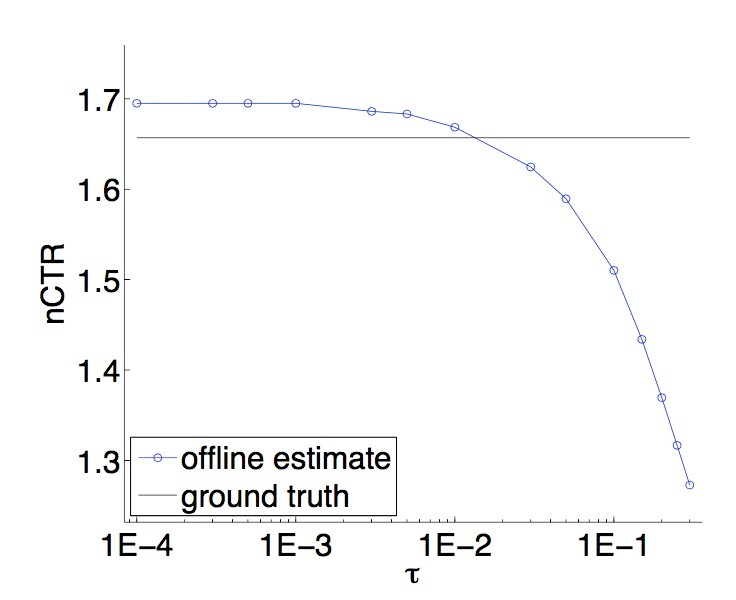## 4.2 实验II

$\hat{V}_{\hat{\pi}}^h = \frac{1}{T} \sum\limits_{t=1}^T \frac{y_t I(h(x_t)=a_t)}{max \lbrace \hat{\pi}(a_t | x_t) , \tau \rbrace}$

…(7)

Interval列的计算使用Chernoff bound相对熵的形式，$\delta=0.05$持有假设：变量是IID的，（即：在我们的case中，用于estimator计算的样本）。注意，该计算有些复杂，因为变量的范围是$[0, 1/\tau]$，而非常见的$[0, 1]$。这可以通过乘以$\tau$来进行缩放，应用到bound上，接着将结果乘以$1/\tau$进行缩放。

“random” policy指的是，从feasible ads集合中随机选择：$Random(x)=a \sim UNIF(C(X))$，其中$UNIF(\cdot)$表示均匀分布。

“Naive” policy对应于理论上有缺陷的监督学习方法（在介绍中详细说过）。这种policy的evaluation相当昂贵，需要每个example每个ad进行一个evaluation，在此，test set的size被减小到一个click 8373个examples，这会减小结果的重要性。通过按时间先后顺序选择在test set中的第一个events（例如：在training set中与它们最相似的events），我们会将该结果偏向于naive policy。然而，naive policy会收到0 reward，这要比其它方法都要小很多。使用这种evaluation的一个可能担忧之处是，naive policy总是会找到那些不会被explored的good ads。一种快速验证表明：这是不正确的——naive argmax会简单地做出难以置信的选择。注意，我们只上报了evaluation against $\tau=0.05$，因为evaluation against $\tau=0.01$并不明显，尽管reward仍是0.

“Learned” policies会依赖于$\tau$。如定理3.2所示，随着$\tau$是减小，我们相竞争的hypotheses的有效集合是增加的，从而允许learned policy具有更好的效果。确实，当我们把$\tau$从0.05减小到0.01时，learned policy和random policy的估计会同时提升。

empirical结果与理论方法大致是consistent的——它们提供了一个一致的关于policy value的最坏估计，不过它们具有足够动态的范围来区分learned polices vs. random policies，以及learned policies在更大空间(更小$\tau$)vs.更小空间(更大$\tau$)上，理论上不完善的naive方法 vs 合理的方法(在ads的explored空间上进行选择)。